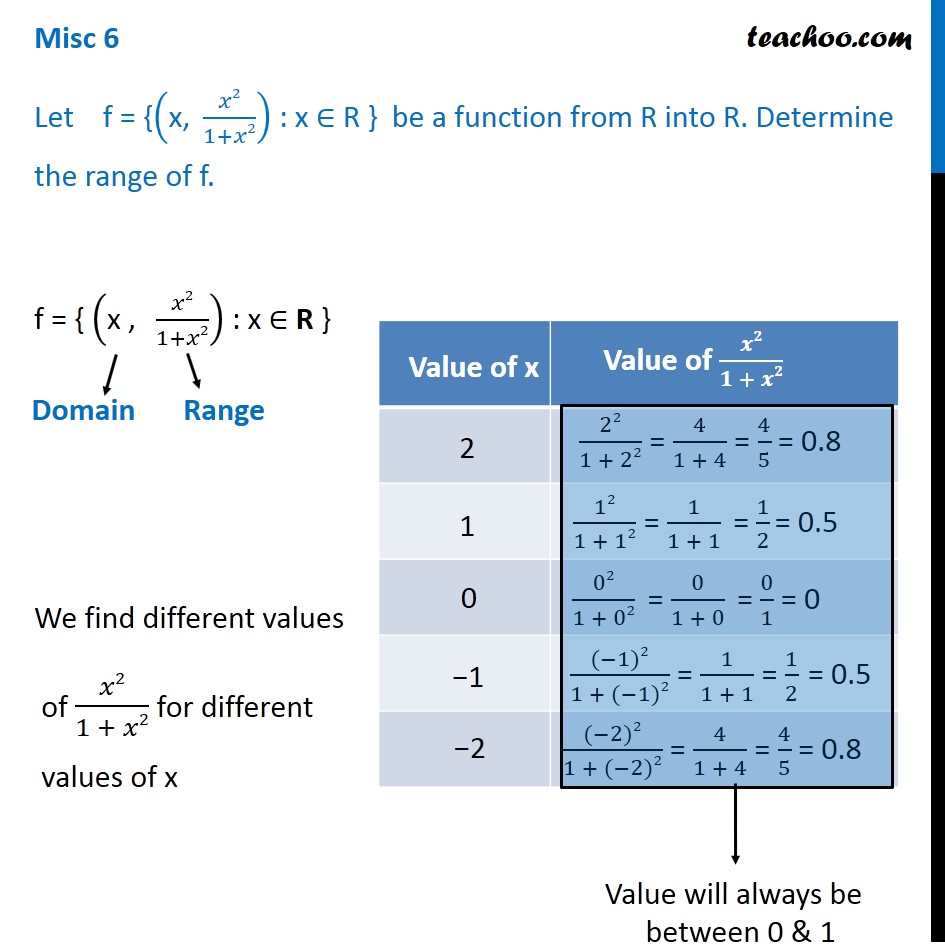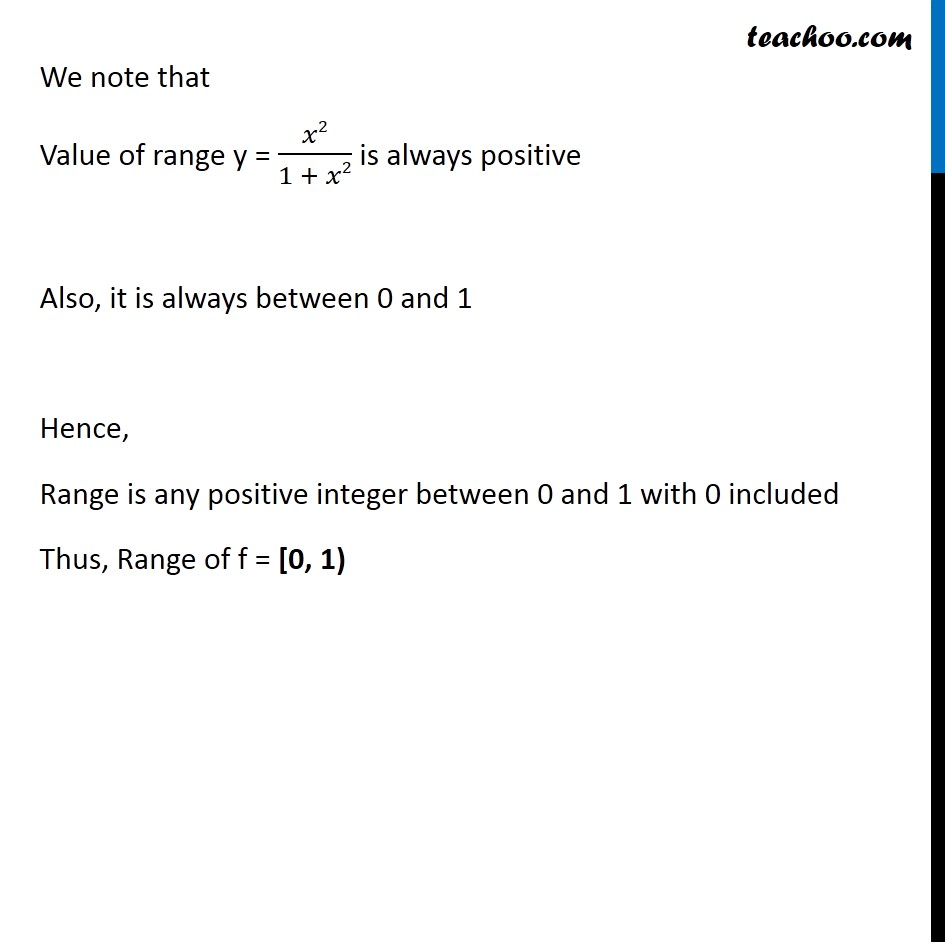Miscellaneous

Chapter 2 Class 11 Relations and Functions
Serial order wiseLearn in your speed, with individual attention - Teachoo Maths 1-on-1 Class

### Transcript

Misc 6 Let f = {("x, " 𝑥2/(1+𝑥2)) : x ∈ R } be a function from R into R. Determine the range of f. f = { ("x , " 𝑥2/(1+𝑥2)) : x ∈ R } We find different values of 𝑥2/(1 + 𝑥2) for different values of x Domain Value will always be between 0 & 1 We note that Value of range y = 𝑥2/(1 + 𝑥2) is always positive Also, it is always between 0 and 1 Hence, Range is any positive integer between 0 and 1 with 0 included Thus, Range of f = [0, 1)